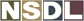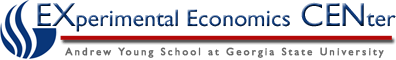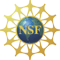Students / SubjectsHandbook >> Demand >>

# Using a Graph

The same information that is presented using words or a table can also be shown on a graph. In economics, we typically use a two-dimensional graph that has the price of the good or service on one axis and the quantity that people are willing and able to buy on the other axis. This way of presenting the information corresponds to the tables above. For example, the information presented in the first table can be shown on a graph as: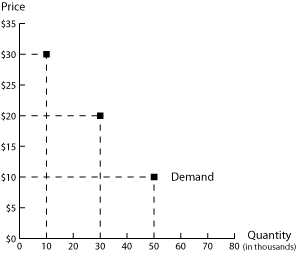Each point on the graph represents a point on the table. The top point shows that when the price of a bottle of red wine is \$30, consumers are willing and able to buy 10,000 cases of wine. Using the terminology presented above, the "quantity demanded" of wine is 10,000 when the price of a bottle of wine is \$30. The middle point shows that the quantity demanded of wine is 30,000 when the price of a bottle is \$20; the bottom point shows that the quantity demanded of wine is 50,000 cases when the price of a bottle of wine is \$10.

Instead of just plotting points on a graph, we usually draw a line through these points to create a more general picture of the demand for the product. We have done this in the next figure: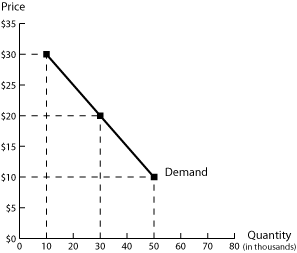Notice that we have the same points as before. But by drawing a line, we showing what we expect consumers to be willing/able to buy at other prices. For example, if the quantity demanded is 50,000 at a price of \$10 and 30,000 at a price of \$20, we might expect consumers to be willing and able to buy 40,000 (half-way between 30,000 and 50,000) when the price of a bottle is \$15 (half-way between \$10 and \$20).
We call this line the "demand curve" (in part because it does not have to be shown as a straight line). The demand curve has a negative slope: this reflects the law of demand. As the price of a product falls, the quantity demanded will increase.

We can also use this demand curve to see the effect of a change in the price of the product: as the price of a bottle of wine falls, we move from the top point on the graph to the middle point on the graph, to the bottom point on the graph. The only effect of a change in the price of the product is to move from one point on the demand curve to another point on the demand curve. So a "change in quantity demanded" is shown on the graph as a movement from one point on a demand curve to another point on the same demand curve.

But what if something else changes? For example, how would we graph the change in tastes and preferences described in the second table above (caused by the release of medical studies showing health benefits of red wine)? We can show this by graphing the three points in the second column of the table.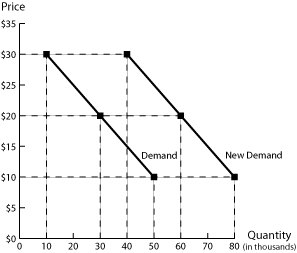Notice that all three points (and the line that joins them) lie to the right of the original line. For example, look at the case where the price of a bottle of wine is \$30; the first demand curve (on the left) shows us that before the studies were released consumers were willing/able to buy 50,000 cases of wine; the second demand curve (on the right) shows us that after the studies were released, consumers were willing/able to buy 80,000 cases of wine. At each point on the second demand curve, consumers are willing/able to buy more wine. This reflects an increase in demand. So a "change in demand" is shown by a shift in the entire demand curve - it requires us to draw an entirely new demand curve.